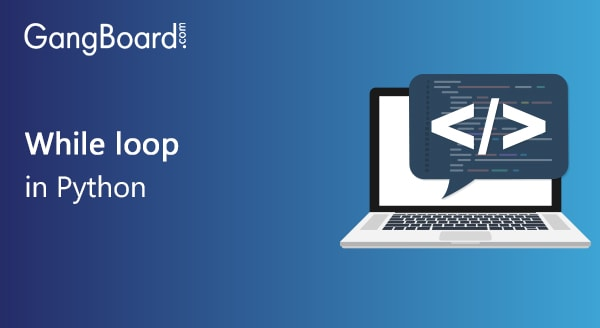# While Loop in Python

January 14th, 2020

## While Loop in Python

Developers use high-level programming languages to code and develop applications that can be either desktop-based or web application based. Python is one such easy to learn scripting language that is very beneficial for developing very intrinsic, scientific applications that require many levels of visualization and data techniques.
A general Python code is made up of functions and methods that perform miscellaneous actions to finally get a single major output. In the world of programming, loops are used by the coders to perform the same set of actions repeatedly for n number of times as set by them.
The most commonly used two kinds of loops in programming are,

• For loop
• While loop
Let us now understand how the while loop works in Python.
The sole concept of the working of the While loop is that the commands specified inside the loop continue to repeat in sequential order as long as the entry condition set for the loop is satisfied.

### Explanation of how the While loop works:

Step 1: As the initial step, the condition specified in the entry of the while loop is checked.
Step 2: If the condition turns FALSE, the control is removed from the loop and the entire operation is terminated then and there. Also, the program control switches from the loop to the next piece of code present sequentially.
Step 3: If the condition turns TRUE, then the set of commands which are nested inside the loop get executed and the control returns to the loop starting for proceeding with the next consecutive iteration.
Now we will explain this with an example.

 while ( i < = 5) print (i) i = i + 1
The output for the above code:
 1 2 3 4 5
There are mainly two different types of iterations as per the number of repetitions. They are

• Definite: In this type of definite iterations, once the entry condition is satisfied, the number of times the code should be performed is mentioned as an integer number by the developer at the start of the loop.
• Indefinite: In this case of indefinite iterations, after the entry criteria are satisfied, the code inside the loop is executed an infinite number of times as there is no limit set by the developer.

### The One-line While Loop

For the purpose of more concise and precise programming, Python also allows its users to write a while loop in a single line to enhance better readability.
Similar to the concept of if statements, while loop in python can also be compressed in a single line. In cases where there are multiple lines of code, they can be separated by the usage of semicolons as delimiters.
Example:

 j = 10 while j < 20: j =  j + 1; print (j)

### Nested While Loop

In special scenarios where one child while loop is placed under a parent while loop, the entire structure is termed as a nested while loop.
Consider the following example for a better understanding

 m = 8 n = 9 while m < 10: while n < 11: print (“Values are: ”,m,n) m = m + 1 n = n + 1
The output for the code will appear like:
 Values are: 8,9 Values are: 9,10

### Usage of else statement:

Python permits its users to benefit from the usage of else statements at the exit of the while loop. The core logic of the ‘else’ statement is that it is used in cases where a pre-defined set of actions can be performed if the entry restriction in the while loop fails.
The code for executing this is as follows,

 while : else :
Here in this code as you can clearly see, the portion of code called the ‘alternate set of actions’ will be executed when the ‘condition to be satisfied’ is failed.

### Usage of break and continue:

Now that you know how to write a while loop in python, the next obvious step is to learn a couple of statements that can alter the flow of the loop. Those statements are:

• Break: The break statement in Python is used to terminate the operation of the loop at a single instance when this statement is encountered.
• Continue: Similarly the continue statement in Python is mainly used to skip executing the current iteration and continue with the further ones in line until the while condition is satisfied.

### Advantages of while loop over for loop:

The main use of ‘while’ loop in Python is the fact that it is used in cases when the developer is not sure of the precise number of times the loop has to be executed.